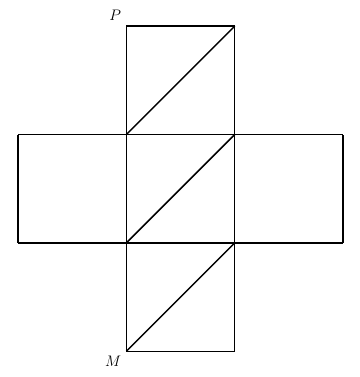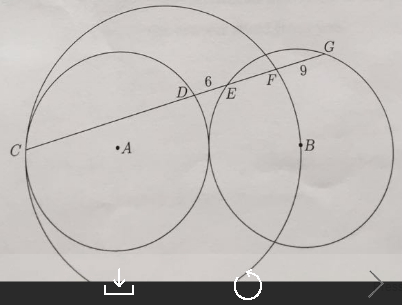###### back to index | new

Solve in integers the equation $$x^2+xy+y^2 = \left(\frac{x+y}{3}+1\right)^3.$$

Quadrilateral $APBQ$ is inscribed in circle $\omega$ with $angle P = \angle Q = 90^{\circ}$ and $AP = AQ < BP$. Let $X$ be a variable point on segment $\overline{PQ}$. Line $AX$ meets $\omega$ again at $S$ (other than $A$). Point $T$ lies on arc $AQB$ of $\omega$ such that $\overline{XT}$ is perpendicular to $\overline{AX}$. Let $M$ denote the midpoint of chord $\overline{ST}$. As $X$ varies on segment $\overline{PQ}$, show that $M$ moves along a circle.

Consider $0<\lambda<1$, and let $A$ be a multiset of positive integers. Let $A_n={a\in A: a\leq n}$. Assume that for every $n\in\mathbb{N}$, the set $A_n$ contains at most $n\lambda$ numbers. Show that there are infinitely many $n\in \mathbb{N}$ for which the sum of the elements in $A_n$ is at most $\frac{n(n+1)}{2}\lambda$. (A multiset is a set-like collection of elements in which order is ignored, but repetition of elements is allowed and multiplicity of elements is significant. For example, multisets ${1, 2, 3}$ and ${2, 1, 3}$ are equivalent, but ${1, 1, 2, 3}$ and ${1, 2, 3}$ differ.)

Point $H$ is the orthocenter of triangle $ABC$. Points $D, E$ and $F$ lie on the circumcircle of triangle $ABC$ such that $AD\parallel BE\parallel CF$. Points $S, T,$ and $U$ are the respective reflections of $D, E, F$ across the lines $BC, CA$ and $AB$. Prove that $S, T, U, H$ are cyclic.

Let $a, b, c$ be positive real numbers such that $a^2 + b^2 +c^2 +(a+b+c)^2 \le 4$. Prove that $$\frac{ab+1}{(a+b)^2}+\frac{bc+1}{(b+c)^2}+\frac{ca+1}{(c+a)^2}\ge 3$$

Let $x$ and $y$ be real numbers such that $$2 < \frac{x-y}{x+y} < 5$$ If $\frac{x}{y}$ is an integer, what is its value?

In how many different ways can 900 be expressed as the product of two (possibly equal) positive integers? Regard $m\cdot n$ and $n\cdot m$ as the same.

A binary palindrome is a positive integer whose standard base 2 (binary) representation is a palindrome (reads the same backward or forward). (Leading zeros are not permitted in the standard representation.) For example, 2015 is a binary palindrome, because in base 2 it is 11111011111. How many positive integers less than 2015 are binary palindromes?

What is the area of region bounded by the graphs of $y=|x+2| -|x-2|$ and $y=|x+1|-|x-3|$?

How many distinct positive integers can be expressed in the form $ABCD-DCBA$, where $ABCD$ and $DCBA$ are 4-digit positive integers? (Here $A, B, C$ and $D$ are digits, possible equal)

In the diagram below, how many different routes are there from point $M$ to point $P$ using only the ling segments shown? A route is not allowed to intersect itself, not even at a single point.In baseball, a player's batting average is the number of hits divided by the number of at bats, rounded to three decimal places. Danielle's batting average is $0.399$. What is the fewest number of at bets that Danielle could have?

Let $n$ be a positive integer. In $n$-dimensional space, consider the $2^n$ points whose coordinates are all $\pm 1$. Imagine placing an $n$-dimensional ball of radius 1 center at each of the $2^n$ points. let $B_n$ be the largest $n$-dimensional ball centered at the origin that does not intersect the interior of any of the original $2^n$ balls. What is the smallest value of $n$ such that $B_n$ contains a point with a coordinate greater than 2?

Say that a rational number is special if its decimal expression is of the form $0.\overline{abcdef}$, where $a, b, c, d, e$ and $f$ are digits (possibly equal) that include each of the digits $2, 0, 1$, and $5$ at least once (in some order). How many special rational numbers are there?

Let $A=(2,0)$, $B=(0,2)$, $C=(-2,0)$, and $D=(0, -2)$. Compute the greatest possible value of the product $PA\cdot PB\cdot PC\cdot PD$, where $P$ is a point on the circle $x^2 + y^2=9$.

A $\textit{permutation}$ of a finite set is a one-to-one function from the set onto itself. A $\textit{cycle}$ in a permutation $P$ is a nonempty sequence of distinct items $x_1, \cdots, x_n$ such that $P(x_1)=x_2$, $P(x_2)=x_3$, $\cdots$, $P(x_n)=x_1$. Note that we allow the 1-cycle $x_1$ where $P(x_1)=x_1$ and the 2-cycle $x_1, x_2$ where $P(x_1)=x_2$ and $P(x_2)=x_1$. Every permutation of a finite site splits the set into a finite number of disjoint cycles. If this number equal to 2, then the permutation is called $\textit{bi-cyclic}$. Computer the number of bi-cyclic permutation of the 7-element set formed by letters "PROBLEMS".

Let $C$ be a three-dimensional cube with edge length 1. There are 8 equilateral triangles whose vertices are vertices of $C$. The 8 planes that contain these 8 equilateral triangles divide $C$ into several non-overlapping regions. Find the volume of the region that contains the center of $C$.

Let $z_1$, $z_2$, $z_3$, and $z_4$ be the four distinct complex solutions of the equation $$z^4-6z^2+8z+1=-4(z^3-z+2)i$$ Find the sum of the six pairwise distance between $z_1, z_2, z_3$ and $z_4$.

An ant begins at a vertex of a convex regular icosahedron (a figure with 20 triangular faces and 12 vertices). The ant moves along one edge at a time. Each time, the ant reaches a vertex, it randomly choose to next walk along any of the edges extending from that vertex (including the edge it just arrived from). Find the probability that after walking along exactly six (not necessarily distinct) edges, the ant finds itself at its starting vertex.

Let $S$ be the sum of all distinct real solutions of the equation $$\sqrt{x+2015}=x^2-2015$$ Compute $\lfloor 1/S \rfloor$.

Let $n$ be a positive integer. When the leftmost digit of (the standard base 10 representation of) $n$ is shifted to the rightmost position (the units position), the result is $n/3$. Find the smallest possible value of the sum of the digits of $n$.

Sabrina has a fair tetrahedral die whose faces are numbered 1, 2, 3, and 4, respectively. She creates a sequence by rolling the die and recording the number on its bottom face. However, she discards (without recording) any role such that appending its number to the sequence would result in two consecutive terms that sum to 5. Sabrina stops the moment that all four numbers appear in the sequence. Find the expected (average) number of terms in Sabrina's sequence.

In the diagram below, the circle with center $A$ is congruent to and tangent to the circle with center $B$. A third circle is tangent to the circle with center $A$ at point $C$ and passes through point $B$. Points $C, A$, and $B$ are collinear. The line segment $\overline{CDEFG}$ intersects the circles at the indicated points. Suppose that $DE=6$ and $FG=9$. Find $AG$.If $P(x)$ denotes a polynomial of degree $n$ such that $P(k) = k/(k +1)$ for $k = 0, 1, 2, \dots n$, determine $P(n + 1)$.

The product of two of the four zeros of the quartic equation $$x^4 - 18x^3 + kx^2 + 200x - 1984 = 0$$ is $-32$. Find $k$.